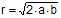Problem 275: Right Triangle, Circumcircle, Sagitta, Inradius Level: High School, SAT Prep, College geometry. In the figure below, ABC is a right triangle inscribed in a circle 1 (circumcircle). If r is the inradius, a is the sagitta of chord BC, and b is the sagitta of chord AB prove that.Recent Additions